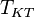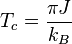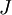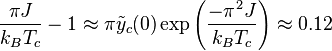# Kosterlitz-Thouless transitionThis article is a 'stub' page, it has no, or next to no, content. It is here at the moment to help form part of the structure of SklogWiki. If you add sufficient material to this article then please remove the {{Stub-general}} template from this page.
The Kosterlitz-Thouless transition (also known as the Berezinskii-Kosterlitz-Thouless (BKT) phase transition)    is a phase transition found in the two-dimensional XY model. Below the transition temperature,$T_{KT}$, the system plays host to a 'liquid' of vortex-antivortex pairs that have zero total vorticity. Above$T_{KT}$ these pairs break up into a gas of independent vortices.$T_c = \frac{\pi J}{k_B}$
where$J$ is the spin-spin coupling constant. This can be obtained as (Eq.58 in ):$\frac{\pi J}{k_BT_c}-1 \approx \pi \tilde{y}_c(0) \exp\left(\frac{-\pi^2J}{k_BT_c} \right) \approx 0.12$